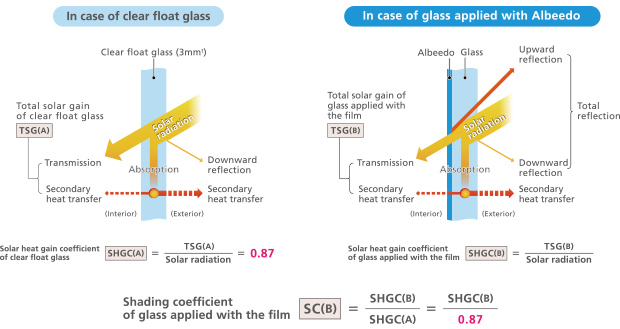# Solar control window film

Solar control window film Albeedo™, improving thermal environment of both inside the building and the street by reflecting near infrared rays upwards.

## Calculated method of window energy efficiency and term definition of optical properties

Solar control window film Albeedo™ has an effect on reduction of solar radiation into a room by reflecting heat rays upward. The reduced solar radiation is equivalent to cooling load reduction of air-conditioner. When you calculate the rough energy improvement effect, please use the calculation formula here below.### Total solar gain (TSG)

TSG of 3mmt clear float glass (TSG (A)) [kWh/m2]
TSG (A) = Solar radiation × SHGC (A) of clear float glass

TSG of glass applied with the film (TSG (B)) [kWh/m2]
TSG (B) = Solar radiation × SHGC (B) of glass applied with the film

• The amount of electricity reduction［kWh］
= { (TSG (A) - TSG (B))［kWh/m2］× total area of the window getting solar radiation［m2］} / COP*
• The amount of money of electricity reduction［\$］
= The amount of electricity reduction［kWh］× unit price of electricity［kWh］
• The amount of CO2 emission reduction［kg-CO2］
= The amount of electricity reduction［kWh］× CO2 emission coefficient［kg-CO2/kWh］
• * COP (Coefficient of Performance)
Ratio of heating/cooling capacity to the effective power input of the air conditioning unit. It is defined as the cooling/heating capacity [kW] divided by rated power consumption [kW].

### Solar heat gain coefficient (SHGC)

The fraction of solar radiation transmitted through a window (glazing), including both directly transmitted part and absorbed and subsequently transferred inward part. TSG is its absolute value. SHGC is the ratio between solar radiation and TSG, and has a value of between 0 and 0.87.

### Shading coefficient (SC)

It is an index of the shading performance of solar radiation. It represents the proportion of solar radiation passes through a 3mm clear float glass applied with a window film as an average criteria 1 compared to that through a plain clear float glass. The lower coefficient value, the better shading performance.

### Visible light transmittance (VLT)

The fraction of total visible light that passes through a window (glazing), weighted by the sensitivity of human eye prescribed by ISO.

### Visible light reflectance (VLR)

The fraction of total visible light that is reflected by a window (glazing), weighed by the sensitivity of human eye prescribed by ISO.

### Ultra-violet rejection (UVR)

The fraction of total ultra-violet light that is rejected from passing through a window (glazing).
UVR = 1 - (UV light transmittance)

### U-value

U-value is an index of heat insulation performance for a glass applied with a window film. It represents how much amount of heat pass per 1m2 in one hour through the 3mm clear float glass applied with a window film under the conditions that air temperature difference between both sides of the glass is exactly 1 degree C. The lower U-value, the better heat insulation performance.

### Near infrared rejection

The fraction of near infrared that is rejected from passing through a window (glazing). The value obtained by calculation assuming the integral value of weighing coefficient of 780-2500nm. Wavelength based on ISO9050 is defined to be 1, and it includes re-emission of near infrared absorbed.

caution

Note on the characteristic data given - Data on the characteristics of the products described in this page based on the results of evaluations carried out by the company. This does not guarantee that the characteristics of the product conform with your usage environment. Before use, review the usage conditions based on evaluation data obtained from the equipment and substrates actually used.